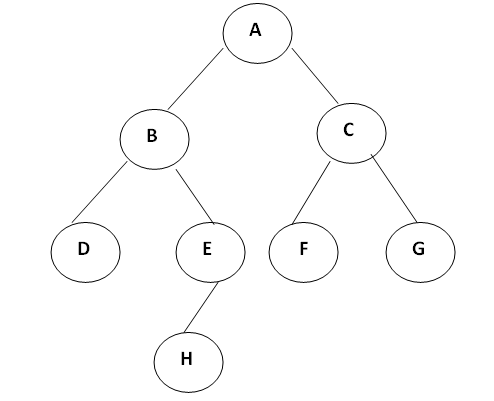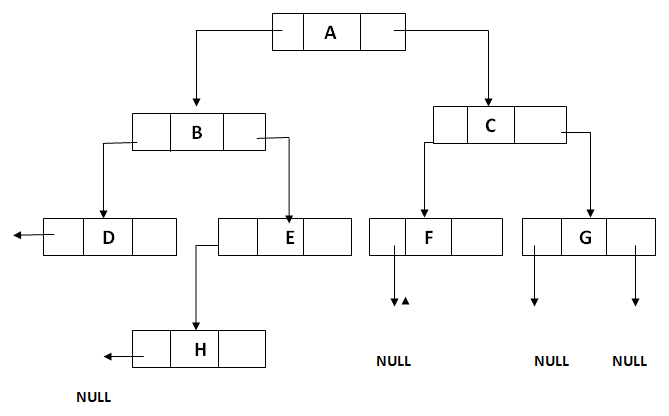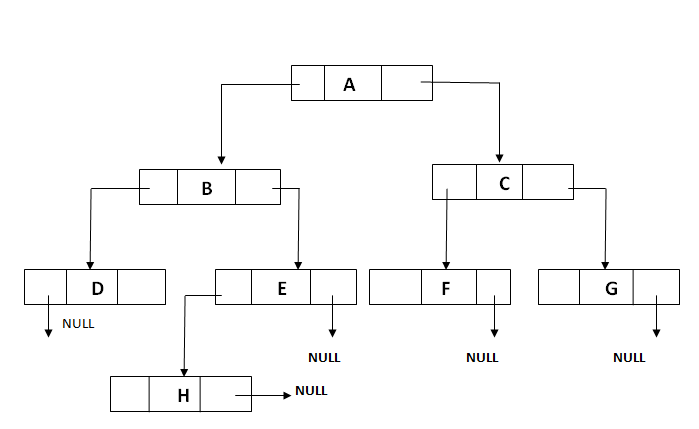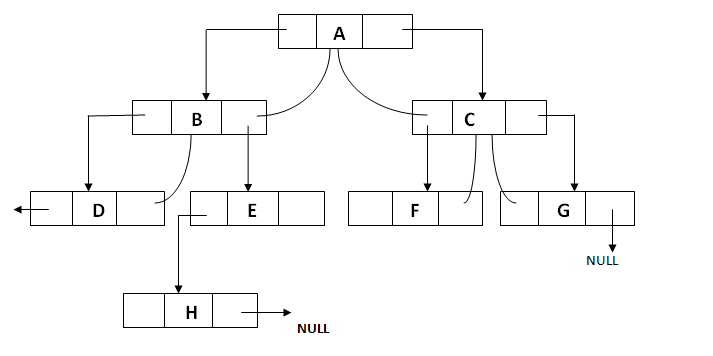# Threaded Binary Tree | Data Structure

Submitted by Prerana Jain, on July 25, 2018

A binary tree can be represented by using array representation or linked list representation. When a binary tree is represented using linked list representation. If any node is not having a child we use a NULL pointer. These special pointers are threaded and the binary tree having such pointers is called a threaded binary tree. Thread in a binary tree is represented by a dotted line. In linked list representation of a binary tree, they are a number of a NULL pointer than actual pointers. This NULL pointer does not play any role except indicating there is no child. The threaded binary tree is proposed by A.J Perlis and C Thornton. There are three ways to thread a binary tree.

1. In the in order traversal When The right NULL pointer of each leaf node can be replaced by a thread to the successor of that node then it is called a right thread, and the resultant tree called a right threaded tree or right threaded binary tree.
2. When The left NULL pointer of each node can be replaced by a thread to the predecessor of that node under in order traversal it is called left thread and the resultant tree will call a left threaded tree.
3. In the in order traversal, the left and the right NULL pointer can be used to point to predecessor and successor of that node respectively. then the resultant tree is called a fully threaded tree.

In the threaded binary tree when there is only one thread is used then it is called as one way threaded tree and when both the threads are used then it is called the two way threaded tree. The pointer point to the root node when If there is no in-order predecessor or in-order successor.

Consider the following binary tree:The in-order traversal of a given tree is D B H E A F C G. Right threaded binary tree for a given tree is shown below:

Non-recursive pre-order, in-order and post-order traversal can be implemented without a stack.

1. Insertion and deletion operation becomes more difficult.
2. Tree traversal algorithm becomes difficult.
3. Memory required to store a node increases. Each node has to store the information whether the links is normal links or threaded links.

### Types of Threaded of Binary Tree

There are three types of Threaded Binary Tree,

Right threaded binary tree for a given tree is shown:Left threaded binary tree for a given tree is shown:Fully threaded binary tree for a given tree is shown: